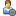###Show Posts

This section allows you to view all posts made by this member. Note that you can only see posts made in areas you currently have access to.

### Messages - The Hanged Man

Pages: 
1
##### Flat Earth Q&A / Re: What makes the sun go around?
« on: September 13, 2008, 12:31:24 PM »
Gravity is a force. If it isn't a force, it isn't gravity.

2
##### Flat Earth Q&A / Re: What makes the sun go around?
« on: September 13, 2008, 10:35:23 AM »
The force of gravity is equal to Gm1m2/R^2 where G is the gravitational constant, m1 is the mass of the one body, m2 is the mass of the second, and R is the distance between the two centers. The force of gravity diminishes in strength proportional to the square of the distance between the two bodies. The Earth is just too small and too far away from our neighbouring planets, mars and venus, to gravitate towards them in any significant way. The moon, however, is close enough.

The acceleration of a body when a force acts upon it is equal to F/m where m is the mass of the body and F is the force acting upon it. The Sun and the Earth gravitate towards each other; however, the sun is so massive that the only apparent acceleration is that of the earth.

I understand how gravitation works, and better than you do, apparently, for there is no force involved. He didn't say that the Earth didn't attract the Sun and other planets in a significant way, he simply stated that it did not attract them, which is untrue.

There is no force involved?

3
##### Flat Earth Q&A / Re: What makes the sun go around?
« on: September 13, 2008, 09:52:42 AM »
The force of gravity is equal to Gm1m2/R^2 where G is the gravitational constant, m1 is the mass of the one body, m2 is the mass of the second, and R is the distance between the two centers. The force of gravity diminishes in strength proportional to the square of the distance between the two bodies. The Earth is just too small and too far away from our neighbouring planets, mars and venus, to gravitate towards them in any significant way. The moon, however, is close enough.

The acceleration of a body when a force acts upon it is equal to F/m where m is the mass of the body and F is the force acting upon it. The Sun and the Earth gravitate towards each other; however, the sun is so massive that the only apparent acceleration is that of the earth.

Pages: